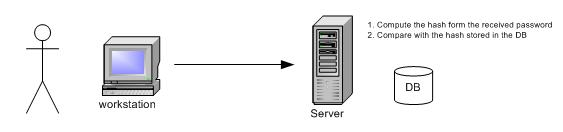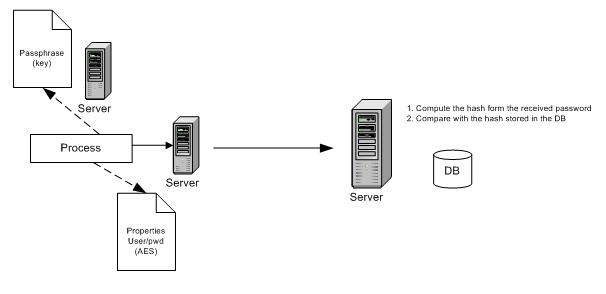##### Transmit a password with MessageDigest
Message digests are secure one-way hash functions that take arbitrary-sized data and output a fixed-length hash value.

A One-way hash function computes a number from a given data. There is no way to decrypt that number to retrieve its original value. The only way is to compute again the same value and check if the result is the same than the previouly computed one.

In this scenario, the user sends a password to the server (through a secure connection). The server computes the computed hash code with the stored hash, if it's the same then the password is correct. The password is never stored in the database.```public class CryptoUtils {

public static void main(String arg[]) {
try {
// quick way to do input from the keyboard, now deprecated...
java.io.StreamTokenizer Input=new java.io.StreamTokenizer(System.in);
//
Input.nextToken();
String hash = byteArrayToHexString(CryptoUtils.computeHash(Input.sval));
System.out.println("the computed hash (hex string) : " + hash);
boolean ok = true;
String inputHash = "";
while (ok) {
System.out.print("Now try to enter a password : " );
Input.nextToken();
inputHash = byteArrayToHexString(CryptoUtils.computeHash(Input.sval));
if (hash.equals(inputHash)){
System.out.println("You got it!");
ok = false;
}
else
System.out.println("Wrong, try again...!");
}
}
catch (Exception e){
e.printStackTrace();
}
}

public static byte[] computeHash(String x)
throws Exception
{
java.security.MessageDigest d =null;
d = java.security.MessageDigest.getInstance("SHA-1");
d.reset();
d.update(x.getBytes());
return  d.digest();
}

public static String byteArrayToHexString(byte[] b){
StringBuffer sb = new StringBuffer(b.length * 2);
for (int i = 0; i < b.length; i++){
int v = b[i] & 0xff;
if (v < 16) {
sb.append('0');
}
sb.append(Integer.toHexString(v));
}
return sb.toString().toUpperCase();
}
}
```
The output is :
```Input your secret password : howto
the computed hash (hex string) : 96A26200CBB466C1DD05CB6D9C7C13F1B90A82AC
Now try to enter a password : Howto
Wrong, try again...!
Now try to enter a password : HOWTO
Wrong, try again...!
Now try to enter a password : howto
You got it!
```
##### Store a password in a properties file
If an automated task needs to log in then you must provide a username/password. Since it's an automated task you can retrieve the username/password from something like a properties file. A security best practice is to never store a password in plain text, you must encrypt it using an appropriate algorithm. The javax.crypto package provides those algorithms and AES is one of them. To retrieve the original value from an encrypted string, you need to use a secret key habitually stored in a different location than the user/password file.

In this scenario, a process gets its user and password from a properties file. The password is crypted, the process uses a "key" stored in a different secure location to decrypt the password. The password is sent to the server for authentication by comparing the computed hash of the received password with the hash code stored for this user in the database.In this HowTo, a key file is created during the encryption process if the specified key file is not found.

```import java.io.File;
import java.io.FileWriter;
import java.io.FileNotFoundException;
import java.io.IOException;
import java.security.GeneralSecurityException;
import java.security.NoSuchAlgorithmException;
import java.util.Properties;
import java.util.Scanner;

import javax.crypto.Cipher;
import javax.crypto.KeyGenerator;
import javax.crypto.SecretKey;
import javax.crypto.spec.SecretKeySpec;

public class CryptoUtils {

public static final String AES = "AES";

/**
* encrypt a value and generate a keyfile
* if the keyfile is not found then a new one is created
* @throws GeneralSecurityException
* @throws IOException
*/
public static String encrypt(String value, File keyFile)
throws GeneralSecurityException, IOException
{
if (!keyFile.exists()) {
KeyGenerator keyGen = KeyGenerator.getInstance(CryptoUtils.AES);
keyGen.init(128);
SecretKey sk = keyGen.generateKey();
FileWriter fw = new FileWriter(keyFile);
fw.write(byteArrayToHexString(sk.getEncoded()));
fw.flush();
fw.close();
}

SecretKeySpec sks = getSecretKeySpec(keyFile);
Cipher cipher = Cipher.getInstance(CryptoUtils.AES);
cipher.init(Cipher.ENCRYPT_MODE, sks, cipher.getParameters());
byte[] encrypted = cipher.doFinal(value.getBytes());
return byteArrayToHexString(encrypted);
}

/**
* decrypt a value
* @throws GeneralSecurityException
* @throws IOException
*/
public static String decrypt(String message, File keyFile)
throws GeneralSecurityException, IOException
{
SecretKeySpec sks = getSecretKeySpec(keyFile);
Cipher cipher = Cipher.getInstance(CryptoUtils.AES);
cipher.init(Cipher.DECRYPT_MODE, sks);
byte[] decrypted = cipher.doFinal(hexStringToByteArray(message));
return new String(decrypted);
}

private static SecretKeySpec getSecretKeySpec(File keyFile)
throws NoSuchAlgorithmException, IOException
{
SecretKeySpec sks = new SecretKeySpec(key, CryptoUtils.AES);
return sks;
}

private static byte [] readKeyFile(File keyFile)
throws FileNotFoundException
{
Scanner scanner =
new Scanner(keyFile).useDelimiter("\\Z");
String keyValue = scanner.next();
scanner.close();
return hexStringToByteArray(keyValue);
}

private static String byteArrayToHexString(byte[] b){
StringBuffer sb = new StringBuffer(b.length * 2);
for (int i = 0; i < b.length; i++){
int v = b[i] & 0xff;
if (v < 16) {
sb.append('0');
}
sb.append(Integer.toHexString(v));
}
return sb.toString().toUpperCase();
}

private static byte[] hexStringToByteArray(String s) {
byte[] b = new byte[s.length() / 2];
for (int i = 0; i < b.length; i++){
int index = i * 2;
int v = Integer.parseInt(s.substring(index, index + 2), 16);
b[i] = (byte)v;
}
return b;
}

public static void main(String[] args) throws Exception {
final String KEY_FILE = "c:/temp/howto.key";
final String PWD_FILE = "c:/temp/howto.properties";

String clearPwd= "my password is hello world";

Properties p1 = new Properties();

p1.put("user", "Real");
String encryptedPwd = CryptoUtils.encrypt(clearPwd, new File(KEY_FILE));
p1.put("pwd", encryptedPwd);
p1.store(new FileWriter(PWD_FILE), "");

// ==================
Properties p2 = new Properties();